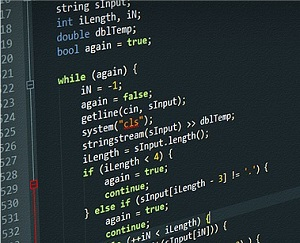We already knew that CoCubes is one of India's leading assessment and hiring platform for IT companies. More than 600+ corporate clients use Cocubes platform to get access to more than a million candidates who already registered and qualified through Cocubes Assessment Test.

Here we are presenting the Cocubes Coding Questions which are important for the candidate's qualification in Assessments Test. In the Skill-based section, candidates are asked questions on the programming capabilities.Cocubes Coding Questions

## Cocubes Coding Questions for Online Assessment Test

Most of the questions in the online assessment test are related to C/ C++ and Java programming.
Questions on C programming, C++ and Java technologies are frequently asked in Cocubes Online Assessment Test. Let's have a look at a few samples and previous years Coding Questions.
• Write a C program to print the numbers from 1 to 100 without using loops
• Write a C program to reverse any number using recursion
• Write a C language program to swap any two numbers without using a third variable
• Write a C program to print Fibonacci series.
• Write a program to print 'N' natural numbers
• Write a C program to check if a given number is a palindrome
• Write a C program to find the factorial of a number using recursion
• Write a C program to find GCD of two numbers using recursion
• Write a C program to find LCM of two numbers using recursion
• Write a C language program for linear search
• Write a C program to find out the sum of digits of a number using recursion
• Write a C program to find the power of a number using recursion
• Write a Java program to print unique characters in a string
• Write a C and Java program to convert the string from upper case to lower case
• Write a C or Java program for concatenation of two strings
• Write a Java program to find out the second largest or second smallest element of an unsorted array
• Write a C++ program to find out the sum of digits of the given number